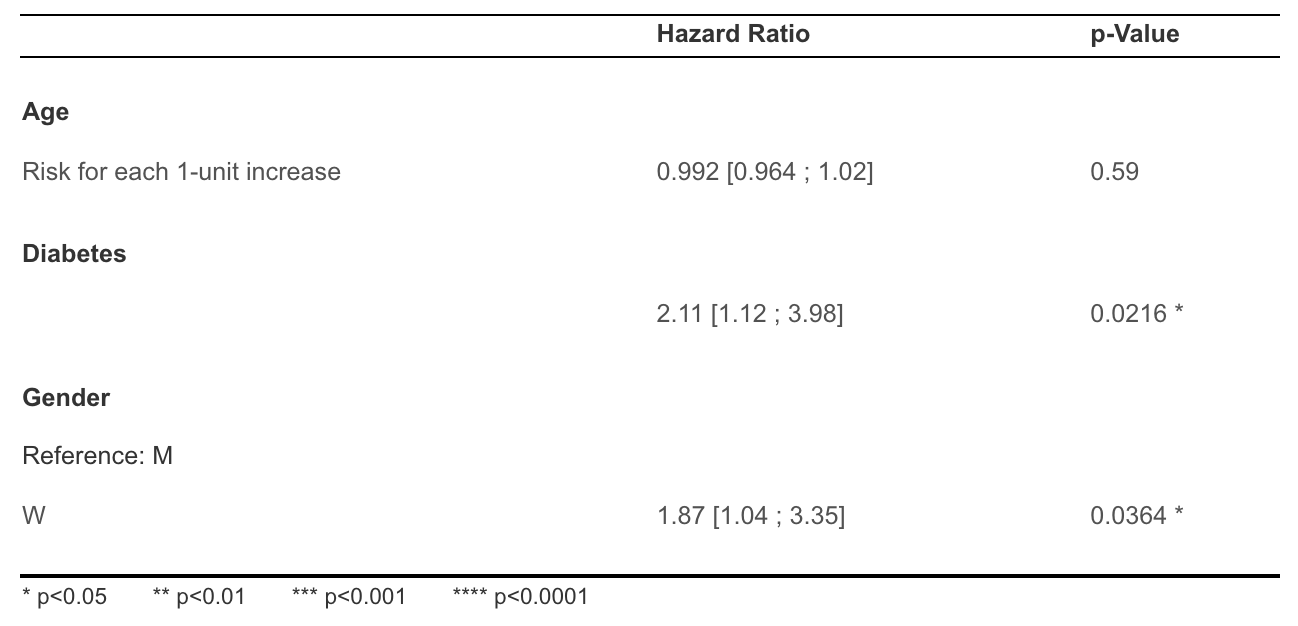# How to calculate hazard ratios (HR) in a Cox model?

Modified on Sat, 04 Dec 2021 at 10:13 PM

# What are hazard ratios?

A Cox model (multivariable survival analysis) allows assessing simultaneously the effect of several factors on survival. It means that you can examine how certain factors influence the rate of a particular event happening (e.g., complication, death, ...) over time. This rate is named hazard rate.

Usually, this hazard rate is expressed as a hazard ratio which is just a mathematical transformation of the hazard rate:

Hazard Ratio = exp( Hazard Rate )

If a predictive variable is associated with a hazard ratio greater than 1, this variable increases the likelihood of the event happening. For example, if smoking is associated with an HR of 2 for the event "cardiovascular disease", it means that smoking increases the risk of this event.

# How to calculate hazard ratios?

You just need to perform a Cox model to calculate HR:

1. In the Statistics menu, click on "Predictive factors (Multivariate)"
2. Select the event variable you want to predict. This could be "Complication date", "Death date", ...
3. Select the variables that you think are predictive and for which you want to calculate the hazard ratio
4. Click on "Calculate results"
5. Head to the results table